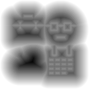Dec 18

#### 尝试在Web上用SDF替代IconFont

Lrdcq , 2020/12/18 15:36 , 程序 , 閱讀(1472) , Via 本站原創

【普通sdf】demo：https://lrdcq.com/test/sdf-canvas/sdf.html

SDF的动态图像生产非常简单：
``````        const d = 50;//边缘检测距离
const sw = image_source.width / 4, sh = image_source.height / 4;
const w = sw + d * 2, h = sh + d * 2;
//原始图片canvas
let canvas_source = document.createElement('canvas');
canvas_source.width = w;
canvas_source.height = h;
canvas_source.style.width = '256px'
canvas_source.style.height = '256px'
document.body.appendChild(canvas_source);

let canvas_source_ctx = canvas_source.getContext('2d');
canvas_source_ctx.drawImage(image_source, d, d, sw, sh);
let canvas_source_data = canvas_source_ctx.getImageData(0, 0, w, h);

//输出sdf的canvas
let canvas_sdf = document.createElement('canvas');
canvas_sdf.width = w;
canvas_sdf.height = h;
canvas_sdf.style.width = '256px'
canvas_sdf.style.height = '256px'
document.body.appendChild(canvas_sdf);

let canvas_sdf_ctx = canvas_sdf.getContext('2d');
let canvas_sdf_data = canvas_sdf_ctx.getImageData(0, 0, w, h);

//遍历所有像素点，找到每个像素点最近边缘
for (let y = 0; y < h; y++) {
for (let x = 0; x < w; x++) {

let scan_left = Math.max(0, x - d), scan_top = Math.max(0, y - d), scan_right = Math.min(w - 1, x + d), scan_bottom = Math.min(h - 1, y + d);
let this_is_1 = !!canvas_source_data.data[(y * h + x) * 4 + 3];
let min_dis = Number.MAX_SAFE_INTEGER;

for (let scan_x = scan_left; scan_x <= scan_right; scan_x++) {
for (let scan_y = scan_top; scan_y <= scan_bottom; scan_y++) {
let that_is_1 = !!canvas_source_data.data[(scan_y * h + scan_x) * 4 + 3];

if (this_is_1 ^ that_is_1) {
let dis = Math.hypot(scan_x - x, scan_y - y);
min_dis = Math.min (min_dis, dis);
}
}
}

min_dis = Math.min(min_dis, d);

canvas_sdf_data.data[(y * h + x) * 4 + 0] = 0;
canvas_sdf_data.data[(y * h + x) * 4 + 1] = 0;
canvas_sdf_data.data[(y * h + x) * 4 + 2] = 0;
canvas_sdf_data.data[(y * h + x) * 4 + 3] = 128 + min_dis / d * 128 * (this_is_1 ? 1 : -1);
}
}``````

SDF的生成原理即找到每个像素点距离矢量图像边缘的距离，生产灰度图。只是如果粗暴的写的话复杂度较高。假设输出sdf长宽w/h，边缘检测距离d，那么一张sdf生产的时间复杂度是O(w * h * d * d)：

- 其实一般sdf生产的图像都会较小，如32x32或者64x64，边缘检测的d考虑到图像拓展和缩放的需求，一般也不会很大，往往8-16即可，上面的代码为了demo演示用了50。
- 另外时间复杂度还有优化空间，毕竟本质是二维图形碰撞测试，用四叉树优化可以裁剪掉50%以上的运算分支，当然这个和图形复杂度相关。
- 精度问题，算出来的灰度的精度，即算出来的距离值约准确约好，因此有多种提升计算精度的方式。a. 这里采用最简单的，扩大计算面积，算一张256x256的sdf再缩小到64x64，精度相当于x4。b. 边缘测量到一个像素内，通过原始的图像像素信息或者矢量信息对边缘部分精确采样，也能更准确的描述边缘。``````        let render = function() {
if (!need_render) {
return;
}
need_render = false;
console.log('Run Render');

canvas_source_ctx.clearRect(0, 0, w, h);
canvas_source_ctx.drawImage(image_source, 0, 0, w, h);
let canvas_source_data = canvas_source_ctx.getImageData(0, 0, w, h);
for (let y = 0; y < h; y++) {
for (let x = 0; x < w; x++) {
canvas_source_data.data[(y * h + x) * 4 + 0] = 0;
canvas_source_data.data[(y * h + x) * 4 + 1] = 0;
canvas_source_data.data[(y * h + x) * 4 + 2] = canvas_source_data.data[(y * h + x) * 4 + 3] >= input2.value ? 255 : 0;
canvas_source_data.data[(y * h + x) * 4 + 3] = canvas_source_data.data[(y * h + x) * 4 + 3] >= input1.value ? 255 : 0;
}
}
canvas_source_ctx.putImageData(canvas_source_data, 0, 0);
}``````

- 注意到只要不是50%的原始位置，有放大或者缩小是图形斜向边缘均会产生波浪形的噪声，这是因为采样缩放算法的误差导致的，并没有很好的办法解决。
- 在图像极其细小的时候图像边缘的粘连现象会十分严重，并且某些区域的缩放情况不符合预期，这里涉及到下一个话题msdf了。

- 对于有缩放的情况，在图像极小或者缩放极的时候，sdf的图像总是圆滑的，即本边缘应该是尖锐的情况也是如此。这个问题的原因是一个尖锐的灰度边缘，在放大后，肯定会变圆，何况我们sdf图的精度往往不够。``````        let render = function() {
if (!need_render) {
return;
}
need_render = false;
console.log('Run Render');

canvas_msdf_ctx.clearRect(0, 0, w, h);
canvas_msdf_ctx.drawImage(image_source_m, 0, 0, w, h);
let canvas_msdf_data = canvas_msdf_ctx.getImageData(0, 0, w, h);
let r, g ,b;
for (let y = 0; y < h; y++) {
for (let x = 0; x < w; x++) {
r = canvas_msdf_data.data[(y * h + x) * 4 + 0];
g = canvas_msdf_data.data[(y * h + x) * 4 + 1];
b = canvas_msdf_data.data[(y * h + x) * 4 + 2];
//rgb三通道叠加两个
value = r + g + b - Math.max(r, g, b) - Math.min(r, g, b);

canvas_msdf_data.data[(y * h + x) * 4 + 0] = 0;
canvas_msdf_data.data[(y * h + x) * 4 + 1] = 0;
canvas_msdf_data.data[(y * h + x) * 4 + 2] = value >= input2.value ? 255 : 0;
canvas_msdf_data.data[(y * h + x) * 4 + 3] = value >= input1.value ? 255 : 0;
}
}
canvas_msdf_ctx.putImageData(canvas_msdf_data, 0, 0);
}``````

- msdf利用多通道，rgba图，视实际情况当然分双通道，三通道，四通道，其中目前基本上三通道够用并且也是主流做法。
- 渲染部分其实也可以分双通道，三通道，四通道，这里是值得，三通道sdf图，如果一个像素在两个通道有值，则叠加有效，上面的demo就是这个做法；或者一个像素在三个通道均有值才有效，这是上面搜到的生成器的做法。者两个做法的区别不大，前者复杂图像边缘可能会有bug只适用于简单icon，只是后者设计于生成难度较大。
- 在msdf来保证精度的情况下，可以缩小sdf图看看最夸张icon图可以小到什么大小。因此demo【迷你msdf】：https://lrdcq.com/test/sdf-canvas/msdf_16.html，采用了几乎不可用的16x16的sdf与msdf。对比来看无论渲染大还是小，msdf的渲染效果均更好。当然整体还是很差，这个尺寸肯定不够用。

【设备：mac pro 15款 chrome，测量方式：得到渲染时间平均值，10次无设备干扰导致的异常值】

- 32x32 10000次：34692 ms
- 64x64 2500次：9045 ms
- 128x128 625次：3010 ms
- 256x256 156.25次：1603 ms

- 渲染的消耗和遍历像素点基本上没啥关系，基本上是固定的，主要消耗在canvas上。也侧面说明纯js计算，就算是O(n*n)也完全不虚。
- 去除最小值，Canvas操作的成本大概是，每156.25次约0.5s左右。也就是说保持60fps的话，canvas同一tick里创建不要超过6个。

- 32x32 1000次：3030 ms
- 64x64 1000次：3561 ms
- 128x128 1000次：4988ms
- 256x256 1000次：9964ms
- 512x512 1000次：30869ms

- 基本上在canvas面积极大的时候，耗时开始跟随面积的指数上升快速上涨了，在前几个值里，成本还是一canvas成本为主，像素计算成本忽略不计

1. SDF/MSDF替代类似于iconfont的功能可行。
2. 相对于font储存，它确实能在更低空间占用的情况下保持矢量优势，并且可以支持较为准确的扩大缩小，可以做艺术字体。当然canvas渲染也可以导出图像。
3. 目前可行的canvas渲染成本较高，大面积使用需要忍受一定掉帧。